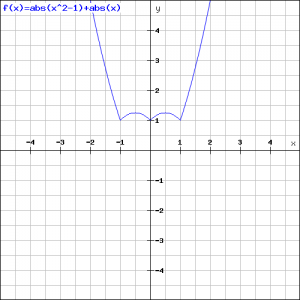# Solution assignment 06 Absolute value |x|

### Assignment 6

Draw the graph of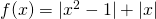### Solution

We apply the definition of the absolute value to both terms.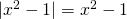if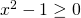, so if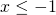or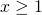(see also assignment 4). Call this interval A.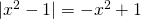if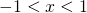. Call this interval B.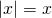if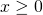. Call this interval C.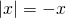if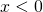. Call this interval D.

To draw the graph we distinguish the following intervals., this is the union of the intervals A and D, briefly AD.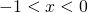, this is the union of the intervals B and D, briefly BD.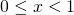, this is the union of the intervals B and C, briefly BC., this is the union of the intervals A and C, briefly AC.

For AD we have: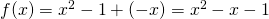For BD we have: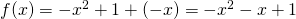For BC we have: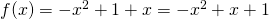For AC we have: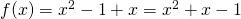The resulting graph is built up by portions of four different parabolas. The first part intercepts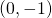and has a minimum for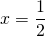. Calculate the same characteristics for the other parabolas and explain them based on the chart. Note that the graph fits the given function.